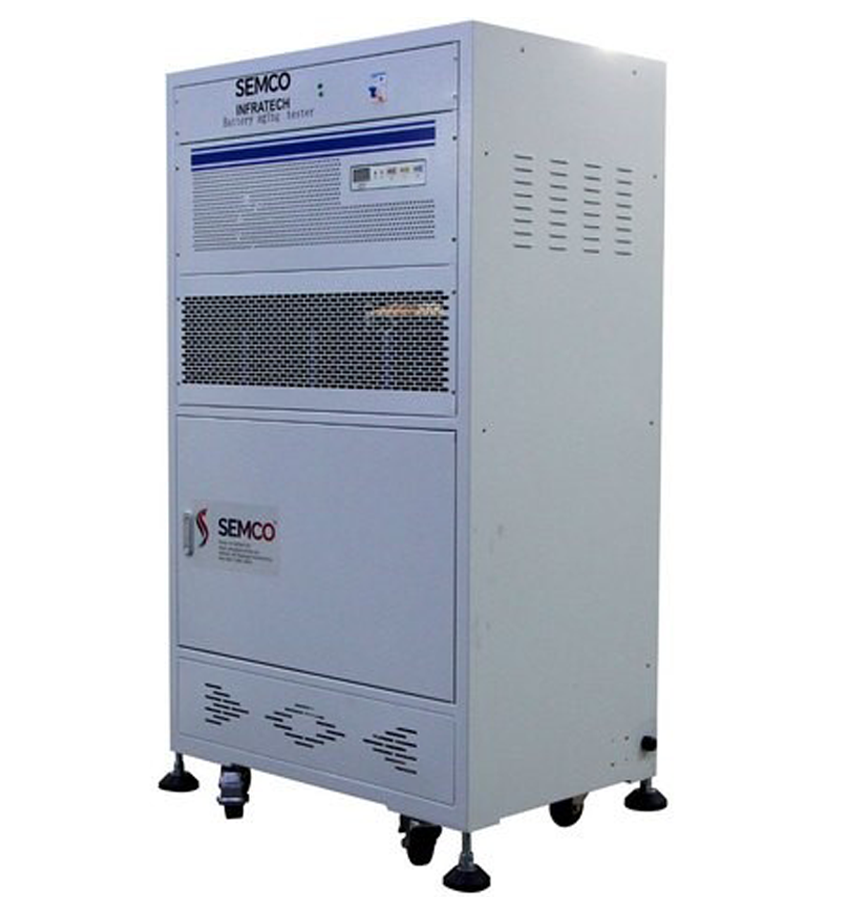Semco university – All about the Lithium-Ion Batteries# Aging Mechanisms of Li-ion Batteries – Semco University##### Introduction

Aging Mechanisms of Li-ion Batteries – The high energy/ power density, and excellent cycle life of the lithium-ion battery have positioned it as the preferred portable energy source for consumer appliances and in the automotive industry. The performance of the battery depends on the development of materials for the various components of the lithium-ion battery. The degradation of these components during battery operation adversely affects the energy delivery of the lithium-ion battery.

• The various battery components undergo different aging mechanisms; the binder and electrolyte decompose, the current collector corrodes, the separator melts and corrodes, and the cathode undergoes structural disorder and metal dissolution.
• The anode undergoes a multitude of aging mechanisms that degrade the electrochemical performance of the lithium-ion battery.

### Sensitivity of Voltage to Capacity

The sensitivity of this experiment refers to the minimum input data required to generate a data reflecting the cell capacity C. The degree of change of the response quantity can be described by the ratio of the response quantity of the instrument or other indicated quantities to the corresponding mass of the measured substance. Specifically, the sensitivity of an analogue resistor, defined as:

The ratio of the capacity Q to the voltage Vk is a very small value, and the battery voltage has no direct relationship with the battery capacity, that is to say, the differential between the voltage and the capacity can be considered as 0. That is to say, the sensitivity is very small on the surface.

Note: SEMCO Battery Charge & Discharge Cabinet Tester SI BCDS 100V 10/20A 7CH.

However, the expansion formula of the voltage includes the open circuit potential, the hysteresis voltage, the capacitive reactance voltage and the impedance voltage, and the factors affecting these voltages include the state of charge Zk, the hysteresis state hk, and the current ik. First analyse the open circuit potential, the differential of the open circuit potential can be expanded by the chain rule as:

The differential of the open circuit potential to the capacity is equal to the product of the differential of the open circuit potential to the state of charge and the state of charge to the capacity. First of all, the derivative of the open circuit potential to the state of charge is very small, that is, the slope of the function is very small, and the contribution to the sensitivity is small. Specifically, as shown in Figure 1:

Secondly, the calculation formula of the state of charge SOC is:

In the measurement experiment, formula (3) is discretized into formula (4) with respect to time:

That is, the state of charge of the current state = the state of charge of the previous time step – the product of the ratio of the time step to the capacity and the Coulomb efficiency of the previous time step and the input current. Therefore, the differential of the state of charge to the capacity can be expressed as:

For the first term to the right of the equal sign, it can be calculated recursively. If the input current ik remains positive, (dZk-1)/dQ will continue to increase after successive iterations, and the value of (dZk -1)/dQ will decrease when ik changes direction. For a random input current ik (for a hybrid electric vehicle), the direction of ik is positive or negative, and the iterative result remains basically the same. At the same time, the denominator of the second term is Q^2 even though the term keeps iterating to a positive value. Since Q must be expressed in Coulomb units for its units to be consistent with the other terms of the equation, Q^2 is a very large number, making the second term very small. Therefore, dZk/dQ is small. The Δt on the molecule is usually converted to 1/3600, which also makes dZk/dQ very small. In summary, the voltage through the OCV term of the open circuit potential contributes very little to the overall sensitivity of the capacity.

Similarly, by analysing the hysteresis voltage, it is found that the sensitivity of the hysteresis voltage hk to the capacity is very small, and the derivation process is omitted here. Therefore, there is very little information about the capacity in the voltage measurement, and the method of measuring the internal resistance cannot be used to measure the battery capacity. It is directly reflected that the mustimeter (voltmeter) cannot detect the capacity, but uses a special capacity detector to measure the capacity.

Note: SEMCO Battery Charge & Discharge Cabinet Tester SI BCDS 99V – 20A – 1CH.

##### Conclusion

The rechargeable lithium-ion battery has been extensively used in mobile communication and portable instruments due to its many advantages, such as high volumetric and gravimetric energy density and low self-discharge rate.

More Articles:

Introduction to Cell Balancing System,
Lithium ion battery fire accidents occur frequently, what is the reason?,
Analysis and summary of lithium battery,
IEC Battery Safety Standard for Power Batteries,
POWER BATTERY SHELL WATERPROOF DESIGN,
Production problems of lithium batteries,